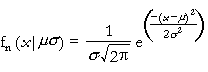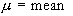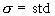Normal Distribution

#Normal Distribution

The Normal Distribution, also called the Gaussian Distribution, is the single most used distribution in applied probability theory. One reason for its popularity is that it can be used to approximate a large variety of distributions in large samples. It's key property is that the sum of a number of normally distributed random variables is also normally distributed. This is extremely useful for variables comprised of additive uncertainties or variables which arise as the sum of a number of random effects (no one of which dominates the total). While the range of applications is extremely broad, some examples are: Population models, insurance payments, the description of error in measurements (or repeated measurements) in biology, physics, astronomy or ballistics. In the oil and gas industry it is used to represent porosity distribution.

In failure analysis the Normal Distribution can be used to model deviations from specified values of manufactured products and constructed items that result from a number of pieces and/or operations (each of which may add some deviation to the total), or the capacity of a system which fails only after saturation of a number of parallel, redundant components has taken place.

The Normal Distribution has a bell shape. It is defined for all values of x, positive and negative. It has two parameters, mu and sigma. The first parameter, mu, is the mean of the distribution and can be any value. The second paramter, s, is the standard deviation (the square root of its variance) of the distribution and must be positive. The distribution is:where sigma>0. (x and mu can have any values.)

The equations for the parameters are:Versions: DPL Professional, DPL Enterprise, DPL Portfolio